# ISEE Middle Level Math : Data Analysis

## Example Questions

### Example Question #151 : Data Analysis

Find the mode of this set of numbers: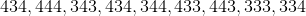Possible Answers: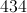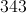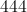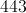Correct answer:Explanation:

The mode is the number that appears in the set most frequently: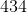Answer: The mode is.

### Example Question #152 : Data Analysis

Find the mode in this set of numbers: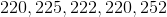Possible Answers: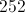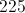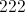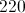Correct answer:Explanation:

The mode is the number the appears most frequently in the data set: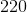### Example Question #153 : Data Analysis

Find the mode in this set of numbers: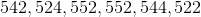Possible Answers: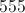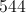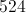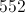Correct answer:Explanation:

The mode is the number in the data set that appears most frequently: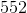### Example Question #154 : Data Analysis

Find the mode in this set of numbers: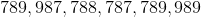Possible Answers: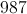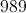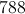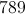Correct answer:Explanation:

Find the most recurring number: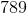### Example Question #155 : Data Analysis

Find the mode in this set of numbers: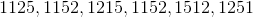Possible Answers: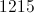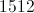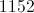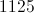Correct answer:Explanation:

Find the most recurring number:### Example Question #156 : Data Analysis

Find the mode in this set of numbers: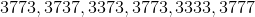Possible Answers: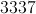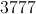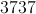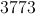Correct answer:Explanation:

The mode is the number in the data set that appears most frequently: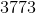### Example Question #157 : Data Analysis

Find the mode of this set of numbers: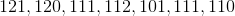Possible Answers: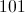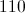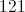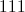Correct answer:Explanation:

The mode is the number that appears most frequently in the data set: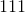### Example Question #158 : Data Analysis

Find the mode in this set of numbers: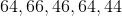Possible Answers: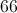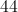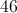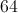Correct answer:Explanation:

The mode is the number that appears most frequently in the data set:### Example Question #159 : Data Analysis

Find the mode in this set of numbers: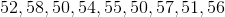Possible Answers: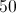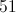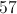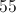Correct answer:Explanation:

The mode is the number that appears most frequently in the data set:### Example Question #160 : Data Analysis

Find the mode in this set of numbers: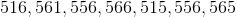Possible Answers: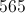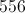Correct answer:Explanation:

The mode is the number that appears most frequently in the data set: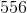### All ISEE Middle Level Math Resources